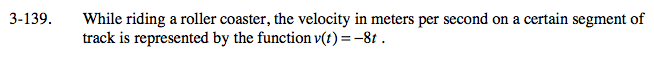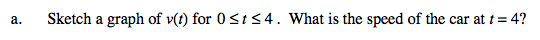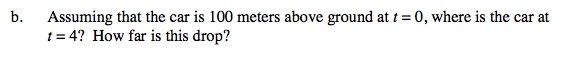### Home > CALC > Chapter Ch3 > Lesson 3.3.4 > Problem3-139

3-139.
1. While riding a roller coaster, the velocity in meters per second on a certain segment of track is represented by the function v(t) = −8t. Homework Help ✎

1. Sketch a graph of v(t) for 0 ≤ t ≤ 4. What is the speed of the car at t = 4?

2. Assuming that the car is 100 meters above ground at t = 0, where is the car at t = 4? How far is this drop?Recall that speed is the absolute value of velocity.

|v(4)| = |− 32| = 32
Don't forget the units!final position = initial position + displacement

intial position is 100 meters

Displacement is area between the velocity curve and x-axis. Notice that the area is negative (below the x-axis).

Total distance of the drop = area under a SPEED graph.